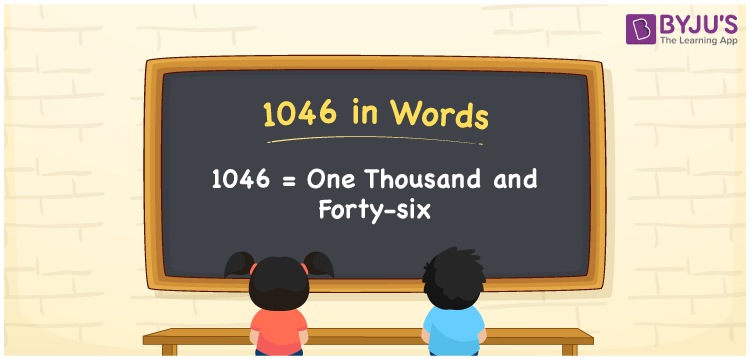# 1046 in Words

1046 in words can be written as One Thousand and Forty-Six. If you buy a motor car for Rs. 1046 for your children, then you can say that “I bought a motor car for One Thousand and Forty-Six Rupees for my children”. Refer to the place value chart given here to write the numbers in words without any confusion. Therefore, 1046 can be read as “One Thousand and Forty-Six” in words.

 1046 in words One Thousand and Forty-Six One Thousand and Forty-Six in Numbers 1046

## 1046 in English Words## How to Write 1046 in Words?

There are four digits in 1046. Students can get to know about the place value of the digits using the chart provided here.

 Thousands Hundreds Tens Ones 1 0 4 6

1046 in expanded form is:

1 x Thousand + 0 × Hundred + 4 × Ten + 6 × One

= 1 x 1000 + 0 × 100 + 4 × 10 + 6 × 1

= 1000 + 40 + 6

= 1046

= One Thousand and Forty-Six

Therefore, 1046 in words is written as One Thousand and Forty-Six.

1046 is a natural number that precedes 1047 and succeeds 1045.

1046 in words – One Thousand and Forty-Six

Is 1046 an odd number? – No

Is 1046 an even number? – Yes

Is 1046 a perfect square number? – No

Is 1046 a perfect cube number? – No

Is 1046 a prime number? – No

Is 1046 a composite number? – Yes

## Frequently Asked Questions on 1046 in Words

### Write 1046 in words.

1046 can be written in words as “One Thousand and Forty-Six”.

### How do you write One Thousand and Forty-Six in numbers?

One Thousand and Forty-Six can be written in numbers as 1046.

### Is 1046 an even number?

1046 is an even number as it is completely divisible by 2.